Courses

# Test: Laws of Exponents

## 20 Questions MCQ Test Mathematics (Maths) Class 9 | Test: Laws of Exponents

Description
This mock test of Test: Laws of Exponents for Class 9 helps you for every Class 9 entrance exam. This contains 20 Multiple Choice Questions for Class 9 Test: Laws of Exponents (mcq) to study with solutions a complete question bank. The solved questions answers in this Test: Laws of Exponents quiz give you a good mix of easy questions and tough questions. Class 9 students definitely take this Test: Laws of Exponents exercise for a better result in the exam. You can find other Test: Laws of Exponents extra questions, long questions & short questions for Class 9 on EduRev as well by searching above.
QUESTION: 1

Solution:
QUESTION: 2

### (64)-3/2

Solution:

(64)-3/2 = ( 8 )2 x - 3/2 =  (8)-3

= 1 / (8)3 = 1/ 512

So option B is correct answer .

QUESTION: 3

### 3x-3  *  5x-4 = 45 What is the value of x?

Solution:

Given,

[3(x-3)] 5(x-4) = 45 = 32 (51)

On comparing both sides we get,

x-3 = 2 and x-4 = 1

=> x = 5

Hence, option A is the correct answer.

QUESTION: 4

Find the value of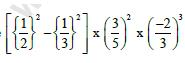Solution:
QUESTION: 5

Which of the following = (100 - 990) x 100?

Solution:
QUESTION: 6

Simplified value of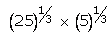is:

Solution:
QUESTION: 7

163/2 is equal to———-

Solution:

(4²)3/2 = (4)3/2 ×2 = (4)³ = 64

QUESTION: 8

Simplify: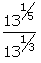Solution:
QUESTION: 9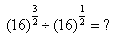Solution:
QUESTION: 10

What is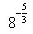equal to?

Solution:
QUESTION: 11

Which of the following is equal to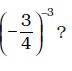Solution:
QUESTION: 12

How many zeros after the decimal point will be there in the decimal form of the number 10-n ?

Solution:
QUESTION: 13

Simplified value of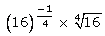is:

Solution:

(16)^-1/4 × 4√16 =(16)^-1/4 × (16)^1/4 =(16)^-1/4+1/4 =(16)^0 = 1.

QUESTION: 14

Which of the following statement is true

Solution:
QUESTION: 15

The value of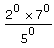is:

Solution:

It's 1 because of a0= 1.
So 1×1/1 = 1

QUESTION: 16

(256)3/4 = ?

Solution: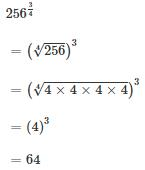QUESTION: 17

What is the value of (-1)-1?

Solution:

(-1)-1 =  - 1 / 1 = -1

QUESTION: 18

811/2 is equal to ——

Solution:

811/2  can be written as (9) 2x1/2 =  9

QUESTION: 19

The value of 491/2 is equal to———

Solution:

491/2 can be written as  (7)2x1/2 = 7

QUESTION: 20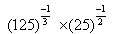=

Solution:

( 5 ) 3 x -1/3   x  ( 5 ) 2  x -1/2

( 5 ) -1  x   ( 5 )  -1

1 / 25